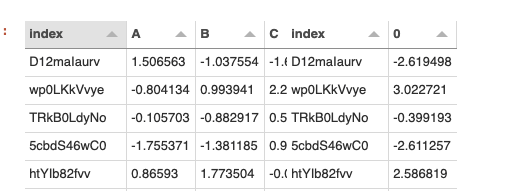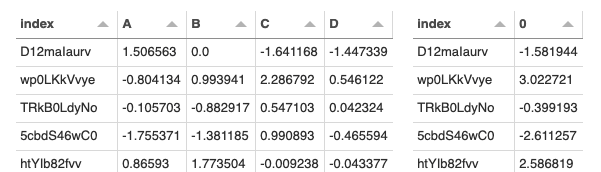# Can I update a view based on a change in a cell in a DataFrame?

What am I doing wrong in the code below? The view_stats does not update when I change values in the dataframe.

``````import param
import pandas as pd
import panel as pn
pn.extension('tabulator')

class Example(param.Parameterized):
dataframe               = param.DataFrame(pd._testing.makeDataFrame())

@param.depends('dataframe', watch=True, on_init=True)
def _update_data(self):
self.stats = self.dataframe.describe()

@param.depends('stats')
def view_stats(self):
return pn.widgets.Tabulator(self.stats)

def view(self):
return pn.widgets.Tabulator(self.dataframe)

e = Example()

pn.Column(e.view_stats, e.view)

``````

Try this: `pn.Column(e.view_stats, pn.Param(e.param, widgets={"dataframe": pn.widgets.Tabulator}))`

1 Like

Awesome, That works. But why does it work?

I think that when you call `pn.widgets.Tabulator` it is disconnected from the class, where `pn.Param` will keep it inside the class.

So the view could look something like this:

``````def view(self):
return pn.Param(self.param, widgets={"dataframe": pn.widgets.Tabulator})
``````

or this

``````def view(self):
return pn.widgets.Tabulator.from_param(self.param.dataframe)
``````

(or just moved up into `__init__`)

2 Likes

Yes I agree with @Hoxbro, @dharhas in your snippet there’s nothing that links the `dataframe` Parameter to its Tabulator widgets. The code is just rendering the dataframe when `view` is called.

There’s also another small issue with this line `@param.depends('stats')`. `stats` isn’t a Parameter, it’s just a regular Python instance attribute, so you can’t depend on it. Param should actually raises an error in this case (this is a small regression after some big improvement done by Philipp on handling dependencies of subparameters, there’s an open issue).

Here’s another approach:

``````import param
import pandas as pd
import panel as pn
pn.extension('tabulator')

class Example(param.Parameterized):

stats = param.DataFrame()

dataframe = param.DataFrame(pd._testing.makeDataFrame())

@param.depends('dataframe', watch=True, on_init=True)
def _update_data(self):
self.stats = self.dataframe.describe()

def view(self):
return pn.Param(self.param, widgets=dict(dataframe=pn.widgets.Tabulator))

e = Example()

e.view()
``````
1 Like

Ok doing this

``````import param
import pandas as pd
import panel as pn
pn.extension('tabulator')

class Example(param.Parameterized):

stats = param.DataFrame()

dataframe = param.DataFrame(pd._testing.makeDataFrame())

@param.depends('dataframe', watch=True, on_init=True)
def _update_data(self):
self.stats = pd.DataFrame(self.dataframe.apply(sum, axis=1))

def view_stats(self):
return pn.widgets.Tabulator(self.stats)

def view(self):
return pn.widgets.Tabulator.from_param(self.param.dataframe)

e = Example()

pn.Row(
e.view,
e.view_stats
)
``````

initially makes the tables overlap until I edit a cell.after I edit a cell the tables move around and I get the followingAlso is there a way to link the indexes?

hmm, maybe this is a bug with Tabulator and pn.Row:

I think it is the same as I reported here: https://github.com/holoviz/panel/issues/3028